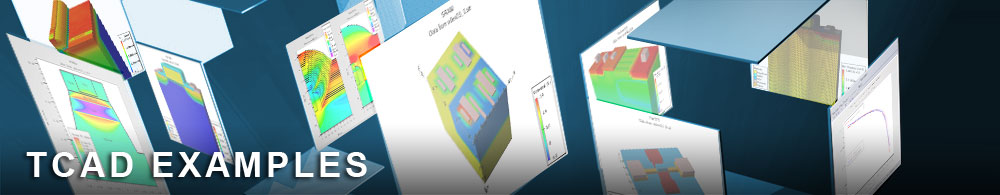Bandstructure

## vaex01.in : Bandstructure

Requires: Victory Atomistic
Minimum Versions: Victory Atomistic 1.0.1.R

This example demonstrates the capability visualizing electronic bandstructures with two methods. The first is the typical plotting along high symmetry points with all the bands shown. The second is for visualizing Ec or Ev in a 2D kspace with maximally localized Wannier function.

The Material section part contains the crystal structures with name "MoS2". The detalied info resides in the all.mat file containing critical dimension for the crystal structure, effective mass or dielectric constants. The hamiltonian file is pointed to by option which is created by wannier90 and post-processed for each atoms having the same orbital number. The readin_atom_xyz_file contains the xyz coordinates of the unit cell with their atomic names indexed if duplicated, e.g. Mo1, Mo@. The number_of_orbitals specified orbital numbers for each atom in the wannier process. The stucture is altogether the intersection of the atomic domain and the Region specified by it's coordinates. In the Domain section, a pseudomorphic domain is created by repetition of a single unit cell with desired repeated number of unit cells.

Schroedinger solves the eigenvalue calculation for the hamiltonian with the Bloch phase due to the periodicity. EK_sample solver calculates eigenvalues over an 2D momentum surface. EK_bulk solver calculates along specific k path, along high symmetry point, designated by {Bold} k_points.

Visualization: 1. contour.R : R-scrip to visualize energy bands along high symmetry points. This can generate a 2D contour plot which shows the conduction band edge for different (kx,ky) coordinates.

{Bold} Use R-interpreter to visualize this file.

2. EK_bulk_k_distance.dat and EK_bulk_energies.dat: Files required to generate E-K plots. {Bold} Use a graph plotter such as Matlab, Python plotter, or Origin to plot the E-k data files.

To load and run this example, select the Load button in DeckBuild > Examples. This will copy the input file and any support files to your current working directory. Select the Run button in DeckBuild to execute the example.